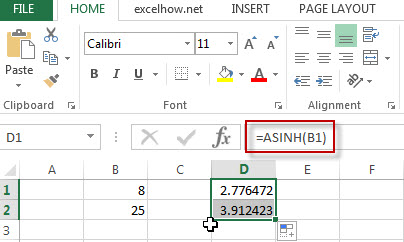# Excel ASINH Function

This post will guide you how to use Excel ASINH function with syntax and examples in Microsoft excel.

### Description

The Excel ASINH function returns the inverse hyperbolic sine of a number.

The ASINH function is a build-in function in Microsoft Excel and it is categorized as a Math and Trigonometry Function.
The ASINH function is available in Excel 2016, Excel 2013, Excel 2010, Excel 2007, Excel 2003, Excel XP, Excel 2000, Excel 2011 for Mac.

### Syntax

The syntax of the ASINH function is as below:

` = ASINH (number)`

Where the ASINH function argument is:

• Number – This is a required argument.  A numeric value

### Excel ASINH Function Example

The below examples will show you how to use Excel ASINH function to return the inverse hyperbolic sine of a number in B1 cell.

#1 = ASINH (B1)Note: the above formula will return the inverse hyperbolic sine of B1 cell.

### Related Functions

• Excel SIN function
The Excel TANH function returns the hyperbolic tangent of a given number.The syntax of the TANH function is as
• Excel ACOS function
The Excel ACOS function returns the arccosine value of a number.The ACOS function is a build-in function in Microsoft Excel and it is categorized as a Math and Trigonometry Function.The syntax of the ACOS function is as below:= ACOS(number)…
• Excel ACOSH function
The Excel ACOSH function returns the inverse hyperbolic cosine of a number.The syntax of the ACOSH function is as below:= ACOSH (number)…
• Excel ASIN function
The Excel ASIN function returns the arcsine value of a number.The syntax of the ASIN function is as below:= ASIN (number)…